# GSEB Solutions Class 11 Maths Chapter 15 Statistics Ex 15.3

Gujarat Board GSEB Textbook Solutions Class 11 Maths Chapter 15 Statistics Ex 15.3 Textbook Questions and Answers.

## Gujarat Board Textbook Solutions Class 11 Maths Chapter 15 Statistics Ex 15.3

Question 1.
From the data given below, state which group is more variable A or B?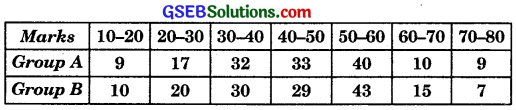Solution: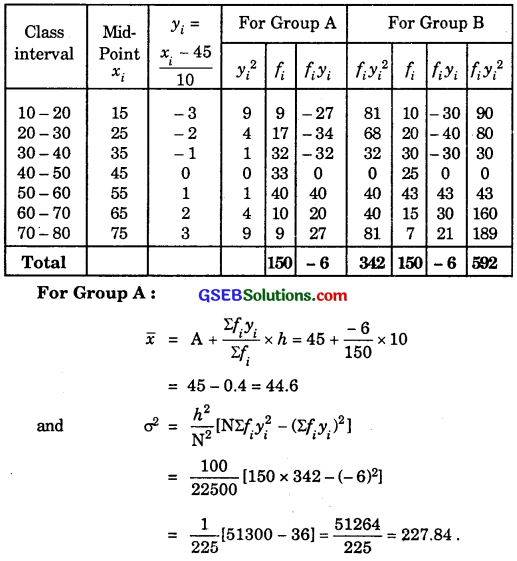∴ σ = 15.09.
Coefficient of variance (C.V.)

For Gropu B:∴ σ = 19.86
Coefficient of variance (C.V.)
= $$\frac{σ}{x}$$ × 100 = $$\frac{19.86}{44.6}$$ × 100 = 44.53.
Coefficient of variation in group B is greater than the coefficient of variation in group A. Therefore, group B is more variable than group A.Question 2.
From the prices of shares X and Y below, find out which is more stable in value: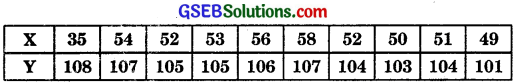Solution: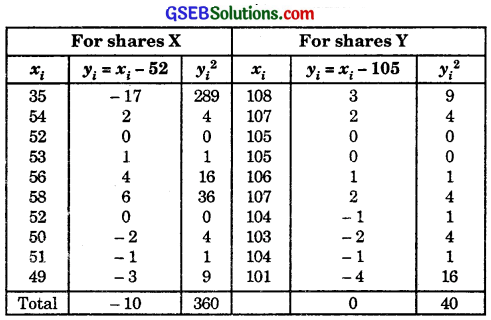For shares X: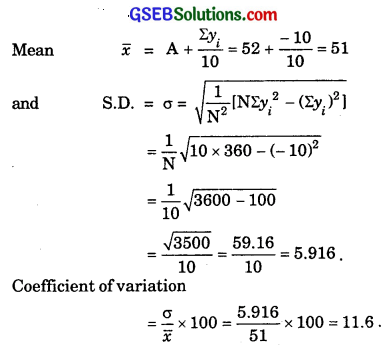For shares Y: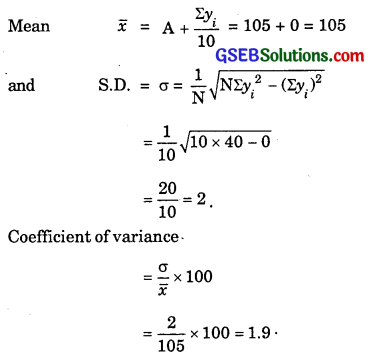Coefficient of variation in shares Y is less than the coefficient of variation in shares X.
Therefore, the share Y is more stable than the share X.Question 3.
An analysis of monthly wages paid to workers in two firms A and B belonging to the same industry, give the following results: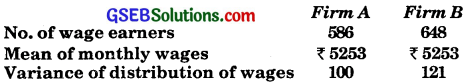(i) Which firm A or B pays out larger amount of monthly wages?
(ii) Which firm A or B, shows greater variability in individual wages?
Solution:
For firm A:
No. of wage earners = 586.
Mean of monthly wages $$\bar {x}$$ = ₹ 5253.
Amount paid by firm A = ₹ (586 × 5253)
= ₹ 3078258.
Variance of distribution of wages = 100.
∴ Standard deviation = σ = $$\sqrt{Variance}$$ = $$\sqrt{100}$$
= 10.
∴ Coefficient of variation = $$\frac{σ}{x}$$ × 100
= $$\frac{10}{5253}$$ × 100 = 0.19.

For firm B:
Number of wage earners = 648.
Mean of monthly wages $$\bar {x}$$ = ₹ 5253.
Amount paid by firm B = ₹ 648 × 5253.
= ₹ 3403944.
∴ S.D. = σ = $$\sqrt{Variance}$$ = $$\sqrt{121}$$
= 11.
∴ Coefficient of variation = $$\frac{σ}{x}$$ × 100
= $$\frac{11}{5253}$$ × 100 = 0.21
Monthly wages paid by firm A = ₹ 3078258.
Monthly wages paid by firm B = ₹ 3403944.
Firm B pays out larger amount as monthly wages.
Coefficient of vanation of wages of firm A = 0.19.
Coefficient of vanation of wages of firm B = 0.21.
Therefore, firm B shows greater variability in individual wages.Question 4.
The following is the record of goals scored by team A in a football session: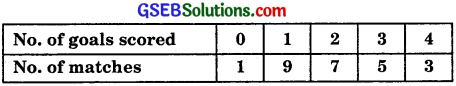For the team B, mean number of goals scored per match was 2 with standard deviation 1.25 goals. Find which team may be considered more consistent?
Solution:For Team A: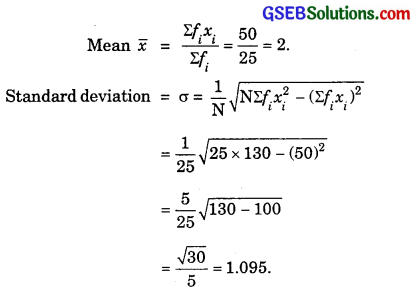∴ Coefficient of variation
= $$\frac{σ}{x}$$ × 100 = $$\frac{1.095}{2}$$ × 100
= 54.75

For Team B:
Mean $$\bar {x}$$ = 2
and S.D. = σ = 1.25.
Coefficient of variation
= $$\frac{σ}{x}$$ × 100 = $$\frac{1.25}{2}$$ × 100
= 62.5.
Coefficient of variation of goals of team A is less than that of B. Therefore, team A is more consistent than team B.Question 5.
The sum and sum of squares corresponding to length x (in cm) and weight y (in grams) of 50 plant products are given below:
$$\sum_{i=1}^{50}$$xi = 212, $$\sum_{i=1}^{50}$$xi2 = 902.8, $$\sum_{i=1}^{50}$$yi = 261, $$\sum_{i=1}^{50}$$yi2 = 1457.6.
Which is more varying, the length or weight?
Solution:
For Length:Coefficient of variation
= $$\frac{σ}{x}$$ × 100 = $$\frac{0.28}{4.24}$$ × 100 = 6.6

For weight: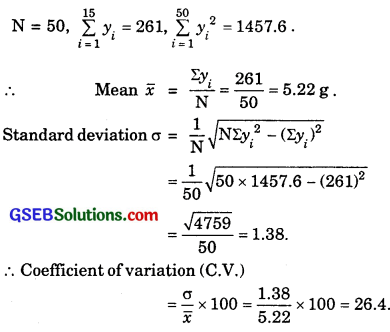Coefficient of variation of weight is more than that of length.
∴ Weight is more varying than length.